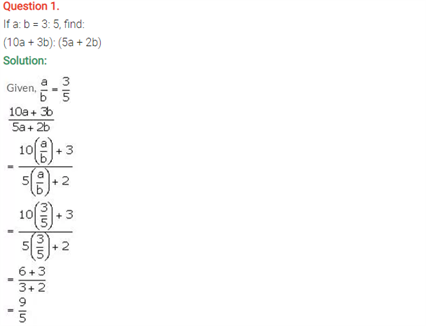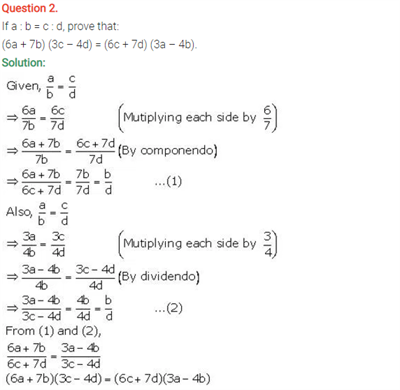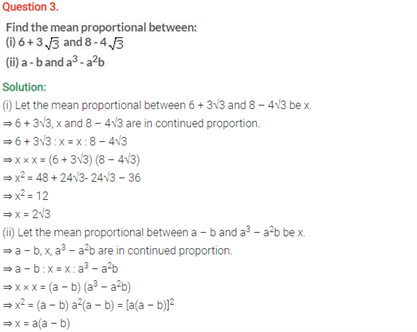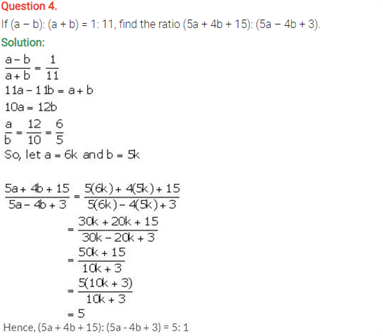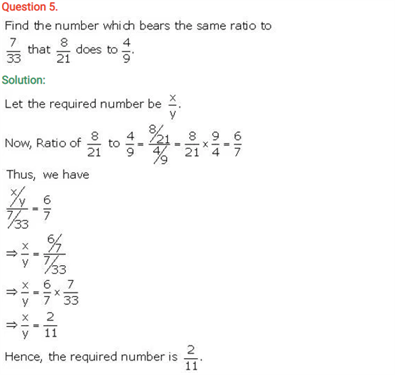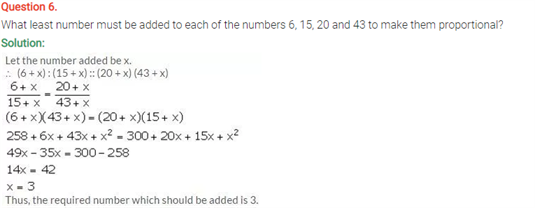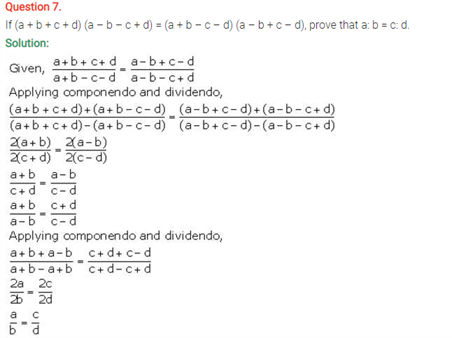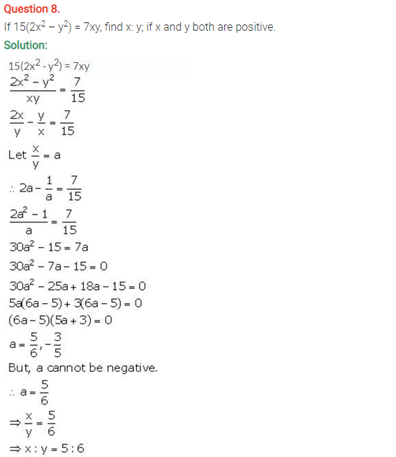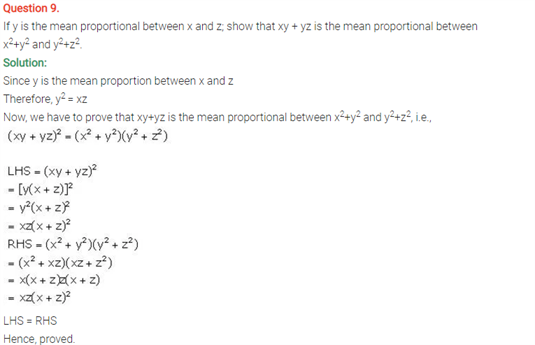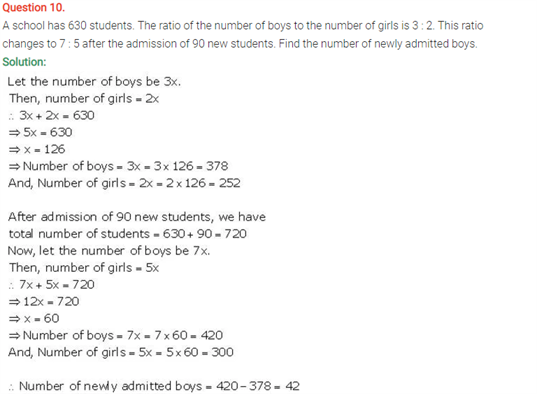# Selina Concise Maths Solution for ICSE Class 10 Chapter 7 Ratio and Proportion (Including Properties and Uses)

## Selina Class 10 Maths Solutions for Chapter 7

We are aware that ratio means the relative size of two quantities expressed as the quotient of one divided by the other and proportion is referred to equality between ratios. Here students will find some more questions on the Ratio and Proportions. Important concepts can be found in the ICSE Solutions For Class 10 Maths and are explained in a comprehensive manner. This chapter has questions that will clear all your doubts regarding this chapter. Lets us see the most basic definitions.

• A ratio is an expression while a proportion is an equation
• Ratios are the comparison of two values of same units and proportion is equating two ratios.
• A ratio is a quantitative relationship between two categories and proportion is the quantitative relationship of a category and the total.

Check out the ICSE Class 10 Maths Selina Solutions Chapter 7 Ratio and Proportion below: